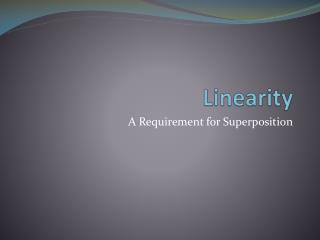DownloadDownload PresentationLinearity

# Linearity

Download Presentation## Linearity

- - - - - - - - - - - - - - - - - - - - - - - - - - - E N D - - - - - - - - - - - - - - - - - - - - - - - - - - -
##### Presentation Transcript

1. Linearity A Requirement for Superposition

2. Objective of Lecture • Introduce the property of linearity • Chapter 4.2

3. Linear Systems • A system is linear if the response (or output) to an input (or excitation) is equal to some constant times the input. Y = f(x) Linear Circuit X Y

4. If x is doubled, Y = f(2x) = 2f(x) If x is multiplied by any constant, a Y = f(ax) = af(x) The system is linear.

5. Linearity • Ohm’s Law is a linear function.

6. Example: DC Sweep of V1

7. I = (1/R1) V1

8. If x = x1 + x2 Y = f(x) = f(x1 + x2) = f(x1)+ f(x2) The system is linear.

9. Mesh Analysis is Based Upon Linearity V3 = 5kW (i1 – i2 ) = 5kW ii – 5kW i2

10. Nonlinear Systems and Parameters Power is nonlinear with respect to current and voltage. As either voltage or current increase by a factor of a, P increases by a factor of a2. P = iv = i2R = v2/R

11. Linear Components • Resistors • Inductors • Capacitors • Independent voltage and current sources • Certain dependent voltage and current sources that are linearly controlled

12. Nonlinear Components • Diodes including Light Emitting Diodes • Transistors • SCRs • Magnetic switches • Nonlinearily controlled dependent voltage and current sources

13. Diode Characteristics An equation for a line can not be used to represent the current as a function of voltage.

14. Example: Find I This circuit can be separated into two different circuits – one containing the 5V source and the other containing the 2A source. When you remove a voltage source from the circuit, it should be replaced by a short circuit. When you remove a current source from the circuit, it should be replaced by an open circuit.

15. I = 5V/10W = 0.5A

16. I = 0A

17. Summary • The property of linearity can be applied when there are only linear components in the circuit. • Resistors, capacitors, inductors • Linear voltage and current supplies • The property is used to separate contributions of several sources in a circuit to the voltages across and the currents through components in the circuit. • Superposition